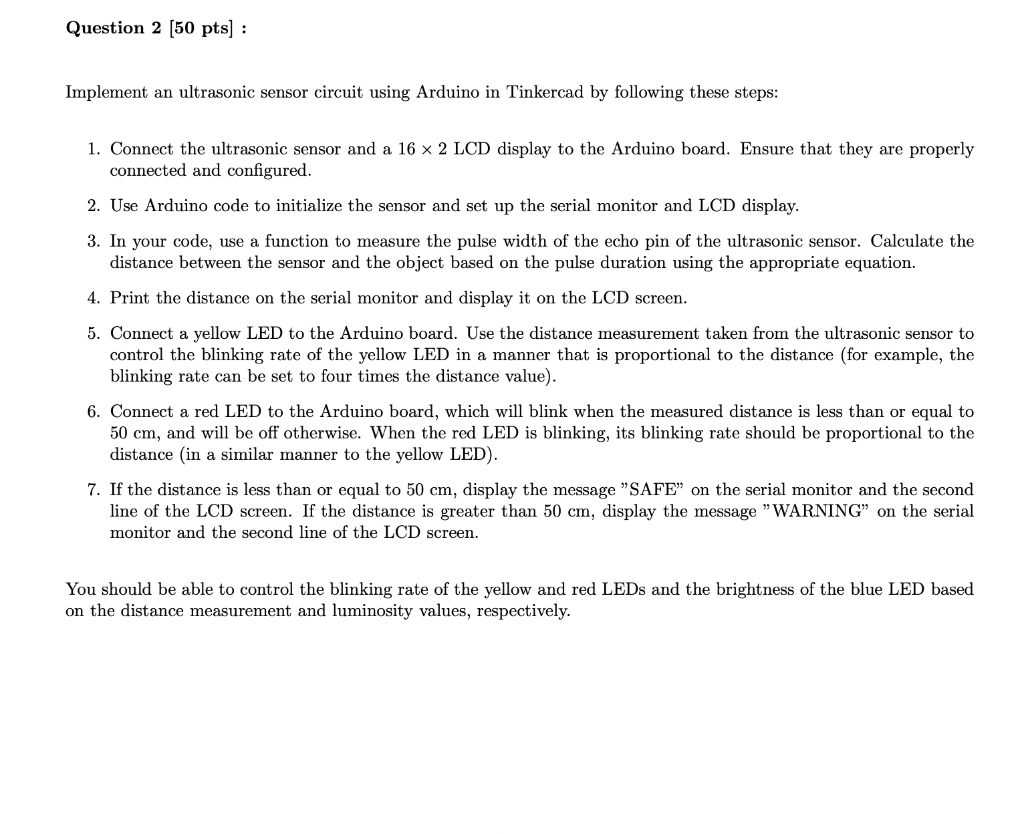# (Solved): Can you add ultrasonic sensor circuit and code screenshoot to solution, plss???   ...

Can you add ultrasonic sensor circuit and code screenshoot to solution, plss??????????

Implement an ultrasonic sensor circuit using Arduino in Tinkercad by following these steps: 1. Connect the ultrasonic sensor and a $$16 \times 2 \mathrm{LCD}$$ display to the Arduino board. Ensure that they are properly connected and configured. 2. Use Arduino code to initialize the sensor and set up the serial monitor and LCD display. 3. In your code, use a function to measure the pulse width of the echo pin of the ultrasonic sensor. Calculate the distance between the sensor and the object based on the pulse duration using the appropriate equation. 4. Print the distance on the serial monitor and display it on the LCD screen. 5. Connect a yellow LED to the Arduino board. Use the distance measurement taken from the ultrasonic sensor to control the blinking rate of the yellow LED in a manner that is proportional to the distance (for example, the blinking rate can be set to four times the distance value). 6. Connect a red LED to the Arduino board, which will blink when the measured distance is less than or equal to $$50 \mathrm{~cm}$$, and will be off otherwise. When the red LED is blinking, its blinking rate should be proportional to the distance (in a similar manner to the yellow LED). 7. If the distance is less than or equal to $$50 \mathrm{~cm}$$, display the message "SAFE" on the serial monitor and the second line of the LCD screen. If the distance is greater than $$50 \mathrm{~cm}$$, display the message "WARNING" on the serial monitor and the second line of the LCD screen. You should be able to control the blinking rate of the yellow and red LEDs and the brightness of the blue LED based on the distance measurement and luminosity values, respectively.

We have an Answer from Expert